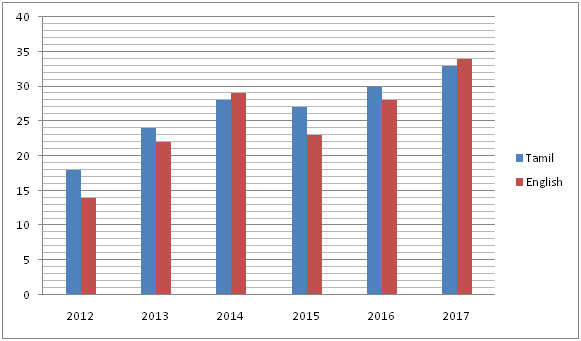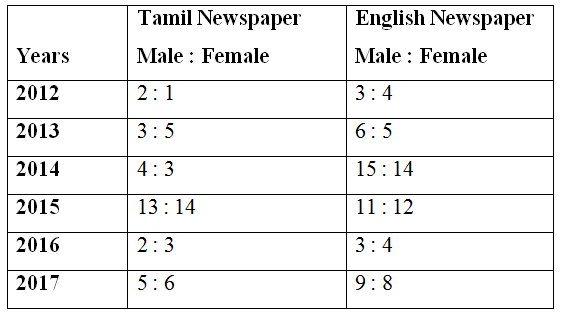# IBPS PO Mains 2018 – Quantitative Aptitude Questions Day-7

Dear Readers, Bank Exam Race for the Year 2018 is already started, To enrich your preparation here we have providing new series of Practice Questions on Quantitative Aptitude – Section. Candidates those who are preparing for IBPS PO Mains 2018 Exams can practice these questions daily and make your preparation effective.

[WpProQuiz 4025]

Directions (Q. 1 – 5): Each question contains a statement followed by Quantity I and Quantity II. Read the contents clearly and answer your questions accordingly.

1) Quantity I: The shopkeeper sold an article at 10 % discount on marked price and he gains 20 %. If the marked price of the article is Rs. 400, then the cost price is?

Quantity II: The shopkeeper marks the price of the book Rs. 350 and his profit % is 15%. Find the cost price of the book, if he allows a discount of 8%?

a) Quantity I > Quantity II

b) Quantity I ≥ Quantity II

c) Quantity II > Quantity I

d) Quantity II ≥ Quantity I

e) Quantity I = Quantity II or Relation cannot be established

2) Quantity I: A and B started a business by Rs. 4800 and Rs. 6400 respectively. A invested only 5 months and they divided their shares after a year. The ratio of profit of A and B is 3 : 8. Then how many months B invested the money?

Quantity II: P and Q invested in the ratio of 5 : 8. P invested the money for 8 months. The ratio of profit of P and Q is 1 : 2. Then, how many months Q invested the money?

a) Quantity I > Quantity II

b) Quantity I ≥ Quantity II

c) Quantity II > Quantity I

d) Quantity II ≥ Quantity I

e) Quantity I = Quantity II or Relation cannot be established

3) Quantity I: Ravi can swim at 10 km/hr in still water. The river flows at 6 km/hr and it takes 6 hours more upstream than downstream for the same distance. How far is the place?

Quantity II: A man can row 20 km/hr in still water and the river is running at 8 km/hr. If the man takes 4 hr to row to a place and back, how far is the place?

a) Quantity I > Quantity II

b) Quantity I ≥ Quantity II

c) Quantity II > Quantity I

d) Quantity II ≥ Quantity I

e) Quantity I = Quantity II or Relation cannot be established

4) Quantity I: The SI on a certain sum of money for 5 years at 8 % per annum is Rs. 7500. Then the principle is?

Quantity II: The CI on a certain sum of money for 2 years at 15 % per annum is Rs. 8800. Then the principle is?

a) Quantity I > Quantity II

b) Quantity I ≥ Quantity II

c) Quantity II > Quantity I

d) Quantity II ≥ Quantity I

e) Quantity I = Quantity II or Relation cannot be established

5) A box contains 7 black balls, 5 pink balls and 4 yellow balls.

Quantity I: If 3 balls are drawn randomly, then the probability of getting at least one pink balls?

Quantity II: If 2 balls are drawn randomly, then the probability of getting both the balls is either black or yellow?

a) Quantity I > Quantity II

b) Quantity I ≥ Quantity II

c) Quantity II > Quantity I

d) Quantity II ≥ Quantity I

e) Quantity I = Quantity II or Relation cannot be established

Directions (Q. 6 – 10) Study the following information carefully and answer the given questions:

The following bar graph shows the total number of Tamil and English newspaper readers (In thousands) in different years in a certain city.The following table shows the ratio of total number of male and female newspaper readers among them.6) Find the ratio between the total number of male Tamil newspaper readers in the year 2012 and 2014 together to that of total number of female English newspaper readers in the year 2013 and 2015 together?

a) 15 : 29

b) 6 : 17

c) 14 : 11

d) 35 : 71

e) None of these

7) Find the difference between the total number of Tamil newspaper readers to that of English newspaper readers in all the given years together?

a) 10000

b) 14000

c) 7500

d) 17500

e) None of these

8) Find the total number of female Tamil newspaper readers in the year 2013, 2015 and 2017 together?

a) 35000

b) 29000

c) 23000

d) 47000

e) None of these

9) The total number of male English newspaper readers in the year 2014 is what percentage of total number of female English newspaper readers in the year 2017?

a) 82.5 %

b) 93.75 %

c) 73.25 %

d) 68.5 %

e) None of these

10) The total number of Tamil newspaper readers in the year 2013 and 2015 together is approximately what percentage more/less than the total number of English newspaper readers in the year 2012 and 2016 together?

a) 47 % less

b) 35 % more

c) 47 % more

d) 21 % less

e) 21 % more

Direction (1-5) :

Quantity I:

Selling price of the article = 400*(90/100) = Rs. 360

Cost price of the article

= > CP*(120/100) = 360

= > CP = 360*(100/120)

= > CP = Rs. 300

Quantity II:

Selling price of the book = 350*(92/100) = Rs. 322

Cost price of the book = 322*(100/115) = Rs. 280

Quantity I > Quantity II

Quantity I:

According to the question,

(4800*5)/(6400*x) = (3/8)

24000/6400x = 3/8

24000*8 = 6400x*3

X = (32400*8)/(6400*3)

X = 10 months

Quantity II:

According to the question,

(5*8)/(8x) = (1/2)

X = 10 months

Quantity I = Quantity II

Quantity I:

x/(10-6) – x/(10+6) = 6

x/4 – x/16 = 6

3x/16 = 6

X= 32 km

Quantity II:

Speed of still water (x) = 20 km/hr,

Speed of stream (y) = 8 km/hr

D = t*[(x2 – y2)/2x]

= > 4*[(202 – 82)/(2*20)]

= > 4*(400 – 64)/40

= > 4*(336/40)

= > 33.6 km

Quantity I < Quantity II

Quantity I:

SI = (P*n*r)/100

7500 = (P*5*8)/100

P = (7500*100)/40 = Rs. 18750

Quantity II:

C.I = P*[(1 + (r/100))2 – 1]

8800 = P*[(1 + 15/100)2 – 1]

8800 = P*[(115/100)2 – 1]

8800 = P*[(23/20)2 – 1]

8800 = P*[(576/400) – 1]

8800 = P*[176/400]

P = (8800*400)/176 = Rs. 20000

Quantity I < Quantity II

Quantity I:

Total probability n(S) = 16C3

Required probability n(E) = 1 – P(none is Pink)

Probability of getting none is Pink balls,

P(E) = n(E)/n(S) = 11C3/16C3

= > 33/112

Required probability = 1 – (33/112) = 79/112

Quantity II:

Total probability n(S) = 16C2

Required probability n(E) = 7C2 or 4C2

P(E) = n(E)/n(S) = 7C2 or 4C2 / 16C2

= > (21 + 6)/120 = 27/120

= > 9/40

Quantity I > Quantity II

Direction (6-10) :

The total number of male Tamil newspaper readers in the year 2012 and 2014 together

= > 18000*(2/3) + 28000*(4/7)

= > 12000 + 16000 = 28000

The total number of female English newspaper readers in the year 2013 and 2015 together

= > 22000*(5/11) + 23000*(12/23)

= > 10000 + 12000 = 22000

Required ratio = 28000 : 22000 = 14 : 11

The total number of Tamil newspaper readers in all the given years together

= > 18 + 24 + 28 + 27 + 30 + 33 = 160 thousands = 160000

The total number of English newspaper readers in all the given years together

= > 14 + 22 + 29 + 23 + 28 + 34 = 150 thousands = 150000

Required difference = 160000 – 150000 = 10000

The total number of female Tamil newspaper readers in the year 2013, 2015 and 2017 together

= > 24000*(5/8) + 27000*(14/27) + 33000*(6/11)

= > 15000 + 14000 + 18000 = 47000

The total number of male English newspaper readers in the year 2014

= > 29000*(15/29) = 15000

The total number of female English newspaper readers in the year 2017

= > 34000*(8/17) = 16000

Required % = (15000/16000)*100 = 93.75 %

The total number of Tamil newspaper readers in the year 2013 and 2015 together

= > 24000 + 27000 = 51000

The total number of English newspaper readers in the year 2012 and 2016 together

= > 14000 + 28000 = 42000

Required % = [(51000 – 42000)/42000]*100 = 21.42 % = 21 % more

Daily Practice Test Schedule | Good Luck

 Topic Daily Publishing Time Daily News Papers & Editorials 8.00 AM Current Affairs Quiz 9.00 AM Current Affairs Quiz (Hindi) 9.30 AM IBPS PO Mains – Reasoning 10.00 AM IBPS PO Mains– Quantitative Aptitude 11.00 PM Vocabulary (Based on The Hindu) 12.00 PM IBPS PO Mains – English Language 1.00 PM IBPS PO/Clerk – GK 3.00 PM Daily Current Affairs Updates 5.00 PM IBPS Clerk Prelims – Reasoning 6.00 PM IBPS Clerk Prelims – Reasoning (Hindi) 6.30 PM IBPS Clerk Prelims – Quantitative Aptitude 7.00 PM IBPS Clerk Prelims – Quantitative Aptitude (Hindi) 7.30 PM IBPS Clerk Prelims – English Language 8.00 PM